Courses

# NCERT Solution, The Fish Tale, class 5, Mathematics Class 5 Notes | EduRev

## Class 5 : NCERT Solution, The Fish Tale, class 5, Mathematics Class 5 Notes | EduRev

The document NCERT Solution, The Fish Tale, class 5, Mathematics Class 5 Notes | EduRev is a part of the Class 5 Course Mathematics for Class 5 (V) - CBSE and NCERT Curriculum.
All you need of Class 5 at this link: Class 5

PAGE No. - 1

Q.1. Do you know any poem about fish?
Ans.
Yes. The poem is written below,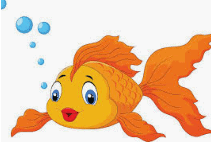One, two, three, four, five.Once I caught a fish alive,
Six, seven, eight, nine, ten,
Then I let it go again.

Why did you let it go?

Because it bit my finger so.

Which finger did it bite?

This little finger on the right.

Q.2. When you think of fishes what shapes come to your mind?
Ans. When I think of fishes, following shapes come to my mind.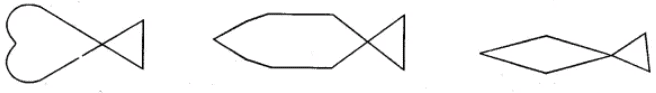Q.3. Try to use a square and a triangle to draw a fish?
Ans. By using a square and a triangle we can draw a fish like below,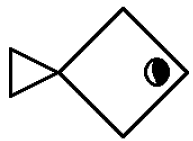PAGE No. - 2

Q.1. ‘Meen’ means a fish and ‘Meenakshi’ is a girl whose eyes look like a fish. Can you think of someone who has such eyes? Draw a face with ‘fish eyes’
Ans. Following is a face, with ‘fish eyes’.Q.2. How long is the biggest fish you can imagine?Ans. One whale shark was as long as 18 m. So, I can imagine that a fish is about 18 metre long.

Q.3. How many times longer is your big fish than the smallest fish?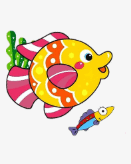Ans. Fishes can have very different sizes.
The smallest fish is about 1 cm long and biggest fish is about 18 m long.
So, 1 m = 100 cm
Then, 18 m = 18 × 100 = 1800 cm.
∴ Big fish is 1800 times longer than the smallest fish.

PAGE No. - 3

Q.1. About how many kilograms do you weigh?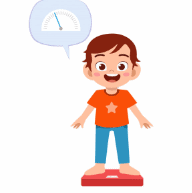Ans. I weigh about 30 kilograms.

Q.2. So, 12 children like you put together will weigh about ______ kg.
Ans. The weight of one child is 30 kg.
Then, Weight of 12 children me put together = 12 × 30 = 360 kg
So, 12 children like me put together will weigh about 360 kg.

Q.3. About how much more does the whale shark weigh than 12 children like you put together?

Ans. Whale shark weight was about 16000 kg.
Weight of 12 children me put together = 360 kg
Then, how much more does the whale shark weigh than 12 children,
= Whale shark weight - Weight of 12 children me put together
= 16000 – 360
= 15,640 kg
∴ The whale shark will weigh 15,640 kg more than the Weight of 12 children me put together.

PAGE No. 5-6

Q.1. How many of you have seen the sea? Where did you see it? Did you see it in a movie or for real? How deep do you think the sea could be? Find out.Ans. I and many of my friends have also seen the sea. I have seen the sea at Mangalore. I have seen the see in a movie and also in real. I think the depth of the sea is more than 500 feet.

Q.2. Do you know how to swim? Would you be scared of the high sea waves?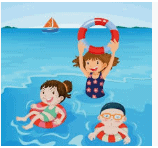Ans. No, I don’t know how to swim. Yes, I scared of the high sea waves.

Q.3. Close your eyes and imagine the sea with waves rising high.
Ans. Yes, I can imagine the sea with waves rising high it was amazing.

Q.4. How high do you think the waves can go?Ans. I think that, the waves can go more than 45 meters.

Q.5. These log boats do not go very far. If the wind is helpful, they travel about 4 km in one hour.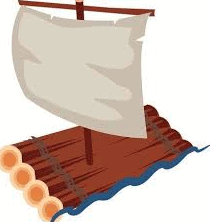(a) How long will they take to go a distance of 10 km?
Ans. These log boats take one hour to travel 4 km,
Then, log boat take two hours to travel = 2 × 4 = 8 km
But in half an hour the log boat will travel = 4/2 = 2km
∴ the total time they take to go a distance of 10 km = 2 and half an hour.
For 2 hours boat travel = 8 km
For 1/2 an hour boat travel = 2 km

(b) Guess how far you can go in one hour if you walk fast.
Ans. I think, I can cover 5 km to 6 km if I walk fast.

PAGE No. - 7

Find out
Q.1. Look at the sun and find out the direction from where it rises.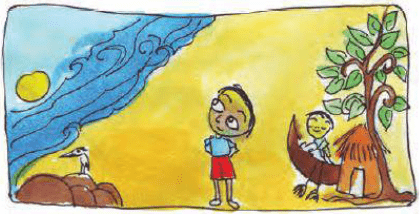(a) From where you are, what interesting thing do you see to your east?
Ans. I can see sun is rising in the east and wind is blowing.

(b) Name two things that are lying to your west.
Ans. Hut and tree are the two thing lying to my west.

Q.2. Look at the different types of boats.
Some boats have motors and go further into the sea. Since they go far out they can catch more fish. These boats travel faster, at the speed of about 20 km in one hour.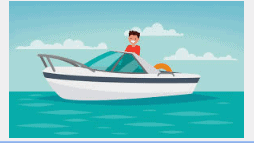(a) How far would the motor boats go in three and a half hours?
Ans. As mentioned in the question these motor boats travel at the speed of about 20 km in one hour.
Then the distance travel by motor boats in three and half an hours
= 20 × 3.5
= 70 km

(b) How much time will they take to go 85 km?
Ans. As mentioned in the question these motor boats travel at the speed of about 20 km in one hour.
Then the distance travel by motor boats in four hours
= 20 × 4
= 80 km
The distance travel by motor boats in 1/4 hour = 1/4 × 20
= 5 km
The total time taken by motor boat to go a distance of 85 km
= Distance/ Speed
= 85/20
= 4 hours 15 minutes.

PAGE No. - 9

Passage: In one trip the log boat brings about 20 kg of fish. But other types of boats bring a bigger catch as given in the table.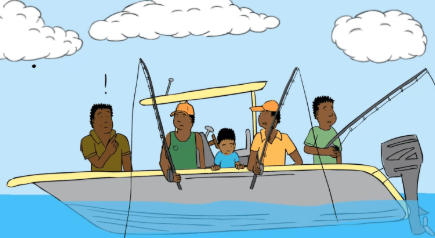The table also shows the speed of each type of boat, which is how far each boat goes in one hour. Look at the table and calculate —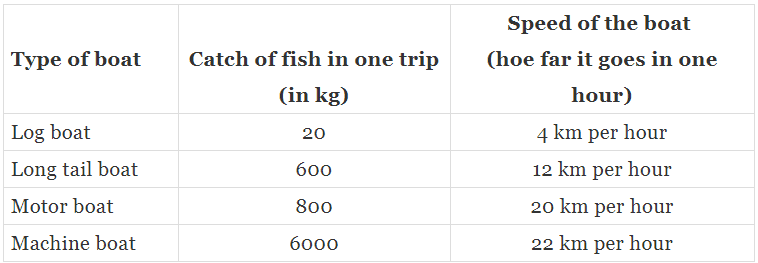(a) About how much fish in all will each type of boat bring in seven trips?
Ans.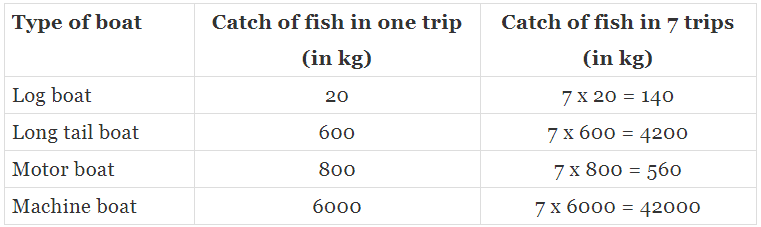(b) About how far can a motor boat go in six hours?
Ans.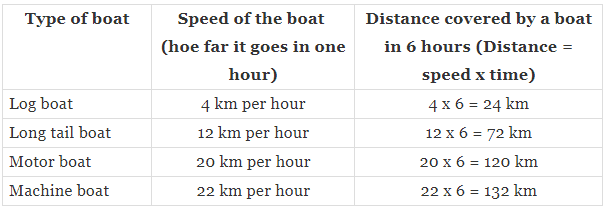(c) If a long tail boat has to travel 60 km how long will it take?
Ans. From the given table long tail boat travel at a speed of 12 km per hour.
So, time taken by the long tail boat to travel 60 km
= distance/speed
= 60 /12
= 5 hours.

PAGE No. - 10

Passage: "In the Class IV Math-Magic you heard of the number which is equal to a hundred thousand. You had read that there are about one lakh brick kilns in our country, where bricks are made".
(a) What other things have you heard of in lakhs?
Ans.
(i) Cost of truck
(ii) Cost of bus
(iii) Population in towns, etc.(b) Write the number one thousand. Now write one hundred thousand. So how many zeroes are there in the number one lakh? Easy, isn’t it?
Ans. One thousand = 1000
One hundred thousand is also called as on lakh = 1, 00, 000
Then total number of zeros in one lakh = 5

(c) There are about two lakh boats in our country. Half of them are without a motor. What is the number of boats with a motor? Write it.
Ans. From the question it is given that, there are about two lakh boats in our country.
Then, half of them are without a motor.
The number of boats with a motor = 2,00,000/2
= 1,00,000 motors.

(d) About one fourth of the boats with a motor are big machine boats. How many thousand machine boats are there? Come on, try to do it without writing down.
Ans. From the question it is given that, about one fourth of the boats with a motor are big machine.
Number of boats = 10,000
1/4 × 10,000 = 25000
Therefore, number of machine boats = 25000

(e) Where have you heard of a crore? What was the number used for?
Ans. I heard of a crore in population of country.
1 crore = 1,00,00,000
Total number of zeros are 7.

PAGE No. - 11

Q.1. At what price per kg did Fazila sell the king fish?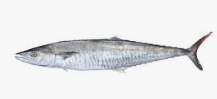Ans. Fazila can hardly carry the big king fish and she says, this fish weighs 8 kg. So, she will sell the whole for ₹ 1200.
Then, the price of that king fish for one kg = 1200/8
= ₹ 150 per kg

Q.2. Floramma has sold 10 kg prawns today. How much money did she get for that?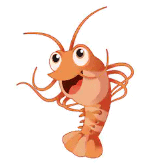Ans. Floramma sells prawns for ₹ 150 a kg.
Given, Floramma has sold 10 kg prawns today.
So, total amount she get = 150 × 10
= ₹ 1500

Q.3. Gracy sold 6 kg sword fish. Mini has earned as much money as Gracy. How many kg of sardines did Mini sell?
Ans. Given, Gracy sold 6 kg sword fish,
Then price of one kg of sword fish = ₹ 60
Total money earned by Gracy
= 6 × 60
= ₹ 360
Mini sold sardines at ₹ 40 per kg.
Total weight of sardines sold by Mini
= 360/40
= 9 kg.

Q.4. Basheer has Rs 100. He spends one-fourth of the money on squid and another three-fourth on prawns.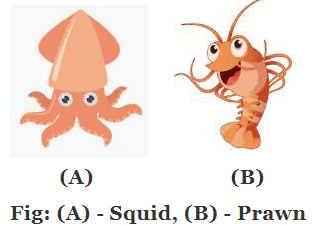(a) How many kilograms of squid did he buy?
Ans. Given, Basheer has ₹ 100
He spends one-fourth of the money on squid = 1/4 × 100
= ₹ 25
Karuthamma sells squid for Rs 50 a kg.
Basheer bought = 25/50 kg
= 1/2 kg of squid.

(b) How many kilograms of prawns did he buy?
Ans. Given, Basheer has ₹ 100.
He spends another three-fourth on prawns = ₹ 75
Floramma sells prawns for Rs 150 a kg.
Basheer bought = 75/150
= 1/2 kg of prawns.

PAGE No. - 12

Passage: Women’s ‘Meenkar Bank’ - The meeting of the Meenkar Bank has just begun. Fazila is the president. Twenty fisherwomen have made their own bank. Each saves Rs 25 every month and puts it in the bank.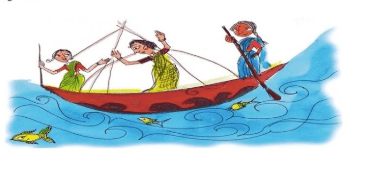Q.1. How much money does the group collect each month?
Ans. There are twenty fisherwomen in the Women’s ‘Meenkar Bank’.
Each saves ₹ 25 every month
So, total money collected in bank each month = 20 × 25
= ₹ 500

Q.2. How much money will be collected in ten years?
Ans. So, from the above solution total money collected in bank per month = ₹ 500
Then, total money collected in bank in one year = 12 × 500
= ₹ 6000
Now, total money collected in bank in 10 years = 6000 × 10
= ₹ 60000

PRACTICE QUESTION
Passage: Gracy needs money to buy a net. Jhansi and her sister want to buy a log boat. So they take a loan from their bank. They will return it with interest.

(a) Gracy took a loan of Rs 4000 to buy a net. She paid back Rs 345 every month for one year. How much money did she pay back to the Bank?
Ans. From the question it is given that,
Gracy took a loan of ₹ 4000 to buy a net.
She paid back ₹ 345 every month for one year.
Then,
Total money she paid in one year to bank = 12 × 345
= ₹ 4,140

(b) Jhansi and her sister took a loan of Rs 21,000 to buy a log boat. They paid back a total of Rs 23,520 in one year. How much did they pay back every month?
Ans. From the question it is given that,
Jhansi and her sister took a loan of Rs 21,000 to buy a log boat.
They paid back a total of ₹ 23,520 in one year.
Then,
Total amount they pay back in every month = ₹ 23,520/12
= ₹ 1,960

PAGE No. - 13

Passage:
"Why Don’t We Start a New Fish-drying Factory? The women of Meenkar Bank also want to start a factory to dry fish. The Panchayat has given them some land for that. Over the years they have saved Rs 74,000. They find out how much they will need for the factory".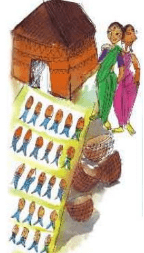Q.1. Fazila writes the things they need to buy to begin. See the table for the cost of each item and the number of items they want to buy. Find the total cost.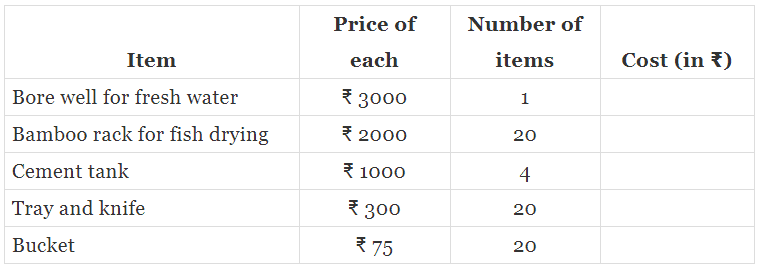Total cost to set up the factory = ____________
When fresh fish is dried it becomes its weight. In one month they plan to dry 6000 kg of fresh fish.
How much dried fish will they get in a month? ____________
Ans.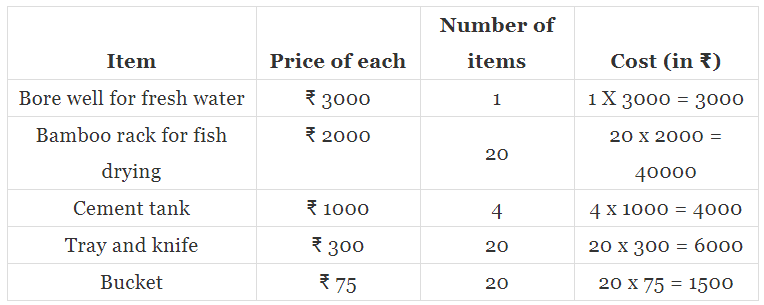Total cost to set up the factory,
= 3000 + 40000 + 4000 + 6000 + 1500
= ₹ 54500
In one month they plan to dry 6000 kg of fresh fish = 1/3 × 6000
= 2000 kg

Q.2. Floramma – let us first calculate for 6 kg of fresh fish.
Example:
We buy fresh fish for ₹ 15 per kg.
We sell dried fish foe ₹ 70 per kg.

(i) We dry 6 kg fresh fish to get _____ kg dried fish
Ans. We dry 6 kg fresh fish to get 2 kg dried fish.

(ii) For 6 kg fresh fish we have to pay 6 × __ = ₹ 90
Ans. For 6 kg fresh fish we have to pay 6 × 15 = ₹ 90

(iii) We will sell 2 kg dried fish and get 2 × __ = ₹ __
Ans. We will sell 2 kg dried fish and get 2 × 70 = ₹ 140

(iv) So if we dry 6 kg fresh fish we will earn __ – 90 = ₹ __
Ans. So if we dry 6 kg fresh fish we will earn 140 – 90 = ₹ 50

(v) But if we dry 6000 kg we can earn ₹ __ × 1000 in one month!
Ans. But if we dry 6000 kg we can earn ₹ 50 × 1000 = ₹ 50000 in one month.

Q.3. Jhansi — I found that for 6000 kg fish we would need 1500 kg salt every month! Its price is Rs ₹ per kg.
Monthly costs:
(a) Salt 1500 × 2 = ₹ ____
(b) Packing and bus charges = Rs 3000
So the total monthly cost of drying and selling the fish = ₹ ____
Fazila — That sounds very good! Our calculations tell us that every month our Bank will earn Rs 44,000!

Ans.
(a) Salt 1500 × 2 = ₹ 3000
Then,
The total monthly cost of drying and selling the fish
= ₹ 3000 + 3000
= ₹ 6000
Hence, earning = 50000 – 6000
= ₹ 44000

Offer running on EduRev: Apply code STAYHOME200 to get INR 200 off on our premium plan EduRev Infinity!

,

,

,

,

,

,

,

,

,

,

,

,

,

,

,

,

,

,

,

,

,

,

,

,

,

,

,

,

,

,

;